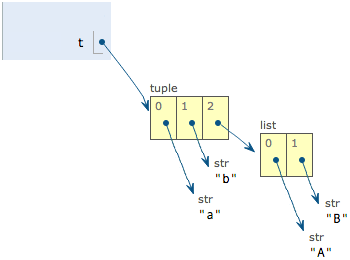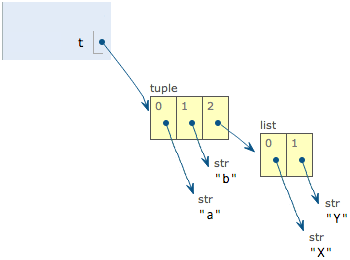# list 和 tuple

`list``tuple` 是 Python 中常用的数据结构。

## # list

Python 内置的一种数据类型是列表: list。list 是一种有序的集合，可以随时添加和删除其中的元素。

``````>>> classmates = ['Michael', 'Bob', 'Tracy']
>>> classmates
['Michael', 'Bob', 'Tracy']
``````

``````>>> len(classmates)
3
``````

``````>>> classmates
'Michael'
>>> classmates
'Bob'
>>> classmates
'Tracy'
>>> classmates
Traceback (most recent call last):
File "<stdin>", line 1, in <module>
IndexError: list index out of range
``````

``````>>> classmates[-1]
'Tracy'
``````

``````>>> classmates[-2]
'Bob'
>>> classmates[-3]
'Michael'
>>> classmates[-4]
Traceback (most recent call last):
File "<stdin>", line 1, in <module>
IndexError: list index out of range
``````

list 是一个可变的有序表，所以，可以往 list 中追加元素到末尾:

``````>>> classmates.append('Adam')
>>> classmates
``````

``````>>> classmates.insert(1, 'Jack')
>>> classmates
``````

``````>>> classmates.pop()
>>> classmates
['Michael', 'Jack', 'Bob', 'Tracy']
``````

``````>>> classmates.pop(1)
'Jack'
>>> classmates
['Michael', 'Bob', 'Tracy']
``````

``````>>> classmates = 'Sarah'
>>> classmates
['Michael', 'Sarah', 'Tracy']
``````

list 里面的元素的数据类型也可以不同，比如:

``````>>> L = ['Apple', 123, True]
``````

list 元素也可以是另一个 list，比如:

``````>>> s = ['python', 'java', ['asp', 'php'], 'scheme']
>>> len(s)
4
``````

``````>>> p = ['asp', 'php']
>>> s = ['python', 'java', p, 'scheme']
``````

``````>>> L = []
>>> len(L)
0
``````

## # tuple

``````>>> classmates = ('Michael', 'Bob', 'Tracy')
``````

tuple 的陷阱: 当您定义一个 tuple 时，在定义的时候，tuple 的元素就必须被确定下来，比如:

``````>>> t = (1, 2)
>>> t
(1, 2)
``````

``````>>> t = ()
>>> t
()
``````

``````>>> t = (1)
>>> t
1
``````

``````>>> t = (1,)
>>> t
(1,)
``````

Python 在显示只有 1 个元素的 tuple 时，也会加一个逗号 `,`，以免您误解成数学计算意义上的括号。

``````>>> t = ('a', 'b', ['A', 'B'])
>>> t = 'X'
>>> t = 'Y'
>>> t
('a', 'b', ['X', 'Y'])
``````## # 小结

list 和 tuple 是 Python 内置的有序集合，一个可变，一个不可变。根据需要来选择使用它们。

• 按正序
• 按倒序
• 按热度